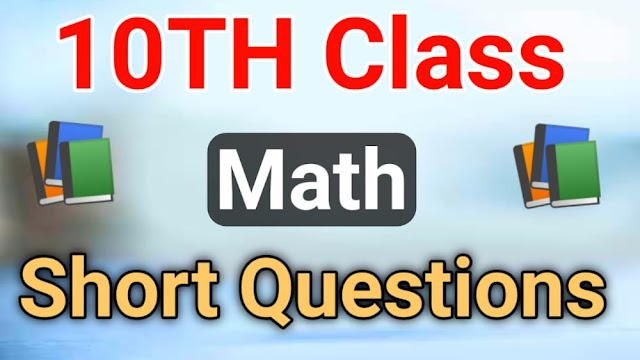# 10th Class Math Important Short Questions - 10TH Class

10th Class, Math, Short Questions, 10th Class Math, Important Short Questions, 10th Class Math Important Short Questions, Class 10 Math Short Question

### Class 10Th Math Short Questions

Math Short Questions Math Short Questions Info
Post TitleMTH603 Midterm Solved MCQs
Book CodeMATH
Current/PastCurrent Math Short Questions For Class 10
Half/FullFull Book Math Short Questions For Class 10
Also, ReadClass 10Th Chemistry Important Short Questions

## 10th Class Math Important Short Questions

These are the 10th Class Math Important Short Questions:

• Write the quadratic equation x2+4/3 - x/7 = 1 in the standard form.
• Solve x2+7x-2=0 using quadratic formula.
• Define reciprocal equation.
• Discuss the nature of roots of equation 2x2-7x+3=0.
• Evaluate (1-3w-3w2)5.
• Without solving, find the sum and product of the roots of quadratic equation 3x2 +7x-11=0.
• Define proportion.
• Find third proportional to 6,12.
• If v=R3 and V=5 when R=3 find R when V=625.
• What is a proper fraction?
• Eresolve 1/x2-1 into partial fractions.
• If X = {1,4,7,9}, Y = {2,4,5,9}, then find YUX.
• Define an onto function.
• If L={a,b,c} , M ={3,4} then find two binary relations of LxM.
• Find the sets X and Y, if XxY= {(a,a),(b,a),(c,a),(d,a)}
• On 5 term tests in mathematics, a student has made marks of 82,93,86,92 and 79. Find the median of the marks.
• Find arithmetic mean by direct method for the following set of data 12,14,17,20,24,29,35,45,
• Define a frequency distribution.
• Define an angle.
• Express the following sexagesimal measure of an angle in decimal form 60,30 30”
• Convert 3r/4 into degrees.
• Find r when l=4cm,0=¼ radians.
• Define projection.
• Define tangent.
• Define the sector of a circle.
• Define triangle.
• Define circumscribed circles.

Students we hope you enjoy after reading these Math Short Questions because these questions are very important according to the final exams point of view and students you know there are all questions we are collected from the final class 10 maths paper for the help of the 10 class students who will search on google then they can easily find our post on the google but don't forget to share these 10th Class Math Short Questions with more students on social media platform where much of 10 class students available for seeking these questions.10th Class Math Important Short Questions

The topic of this post is 10th-Class-Math-Important-Short-Questions and we add this post to 10TH Class category and uploaded many study solutions only for the class 10 students. But if you are in another class then open the other categories related to class code because we made many categories on our website and also added the latest and old study solutions posts for the class 10 students.